# Roman Numerals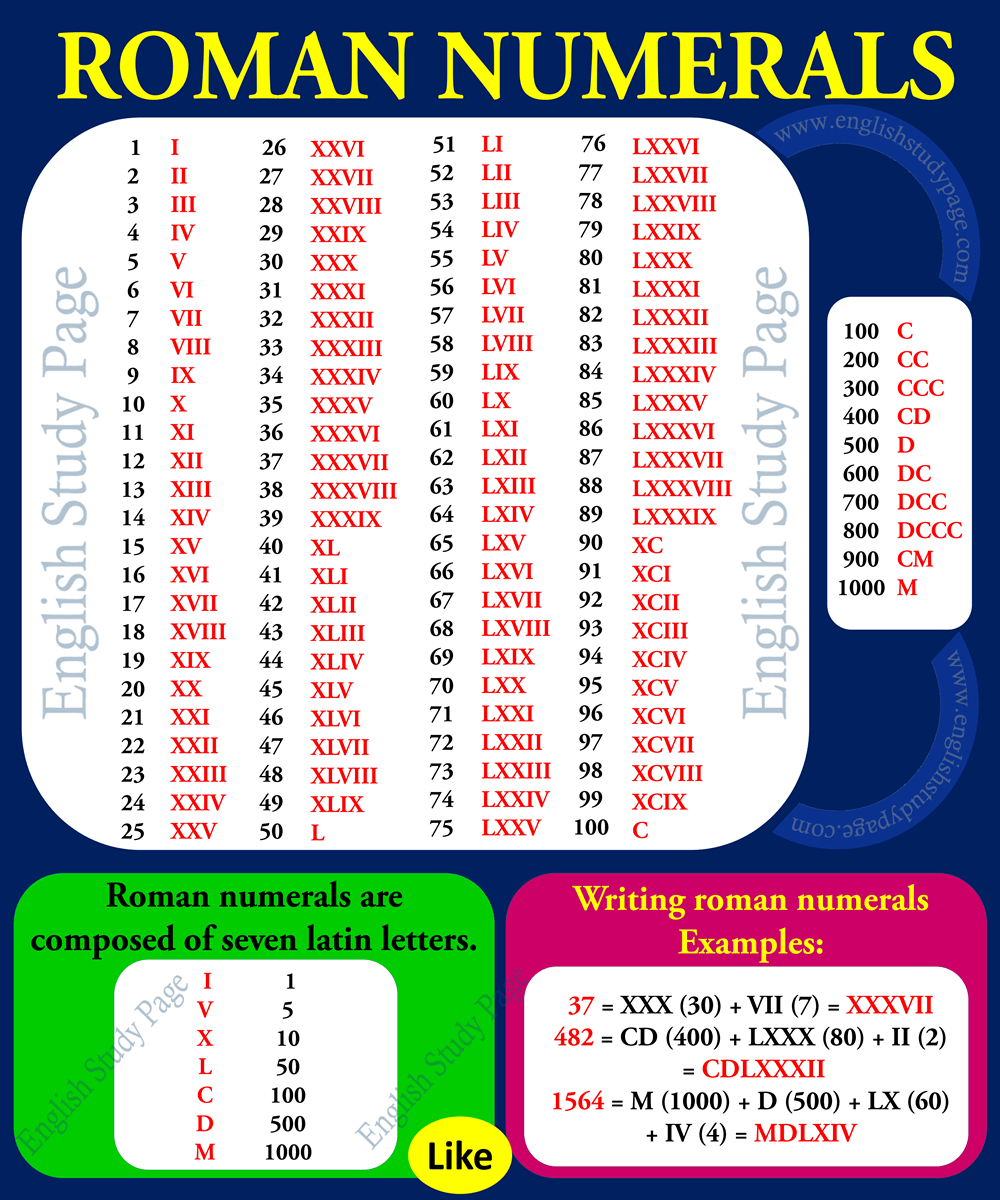Roman numerals, writing roman numerals, etc… Please follow the list for detailed expressions, lists and examples;

A numeral system is a writting system for expressing numbers.

We generally use 10 digits ( 012, 3, 4, 5, 6, 7, 8, 9 ) for writting a number in many parts of the world which are called arabic numbers or arabic numerals.

However sometimes we can use or see another numeral system for writting numbers which are used by the ancient Romans to write numbers ( like I=1, III=3, V=5, X=10, C=100) which are called roman numbers or roman numerals.

 The Letters Used in Roman Numerals ( from lower to higher ) I  =  1 V  =  5 X  =  10 L  =  50 C  =  100 D  =  500 M  =  1000

NOTE 1:

Roman numerals are essentially known as uppercase letters: I, V, X, L, C, D and M.

However, it is possible to use lowercase letters: i, v, x, l, c, d and m.

NOTE 2:

We can make or see a combination is made of smaller groups of these letters ( such as XX – xx, LXVI – lxvi, CCCVII – cccvii), each group in increasing order from right to left. The whole combination represents a number.

Examples:

• XX = X (10) + X (10) = 20
• LXX= L (50) + XX (20) = 70
• CXCII = C (100) + XC (90) + II (2) = 192
• MDLXXXIV = M (1000) + D (500) + LXXX (80) + IV (4) = 1584

NOTE 3:

The letters can be repeated three times to increase the value but the letter can not be repeated more than three times.

It means;

• XXX ( CORRECT )
• XXXX ( INCORRECT)
• CCC ( CORRECT )
• CCCC ( INCORRECT)

You can see some important or most used numbers or numerals below;

 ROMAN NUMERAL ROMAN NUMERAL ARABIC NUMERAL Upper-case Lower-case ———————— I i 1 II ii 2 III iii 3 IV iv 4 V v 5 VI vi 6 VII vii 7 VIII viii 8 IX ix 9 X x 10 XI xi 11 XII xii 12 XIII xiii 13 XIV xiv 14 XV xv 15 XVI xvi 16 XVII xvii 17 XVIII xviii 18 XIV xiv 19 XX xx 20 XXX xxx 30 XL xl 40 L l 50 LX lx 60 LXX lxx 70 LXXX lxxx 80 XC xc 90 C c 100 CC cc 200 CCC ccc 300 CD cd 400 D d 500 DC dc 600 DCC dcc 700 DCCC dccc 800 CM cm 900 M m 1000

From 1- 10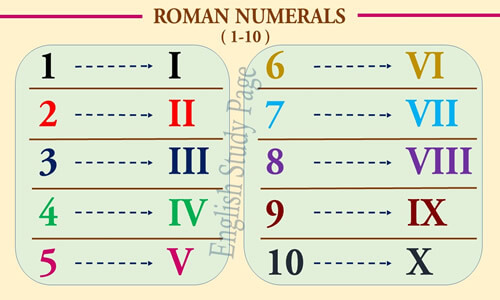From 11 to 20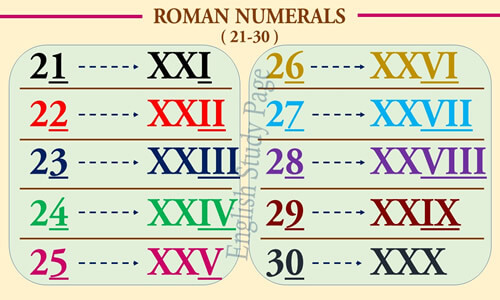From 21 to 30From 31 to 40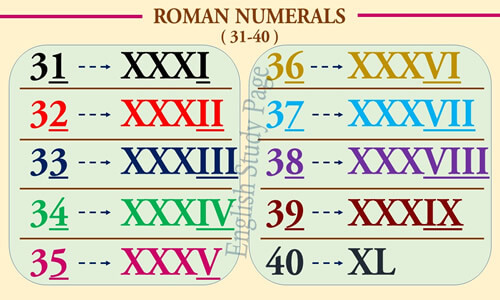From 41 to 50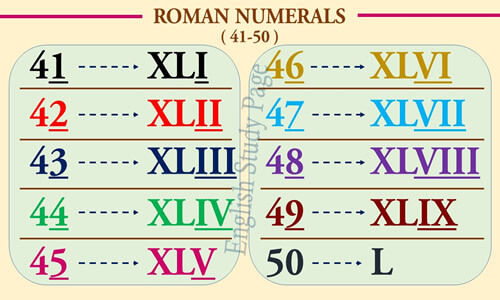From 51 to 60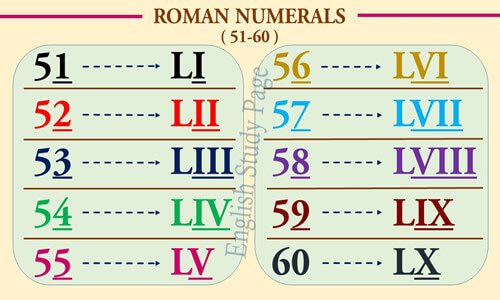From 61 to 70From 71 to 80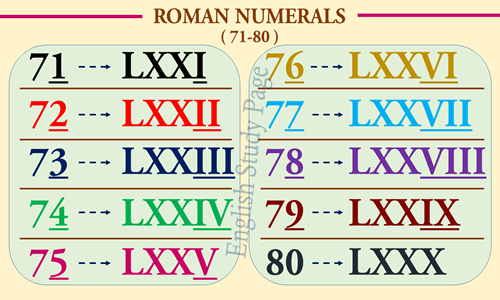From 81 to 90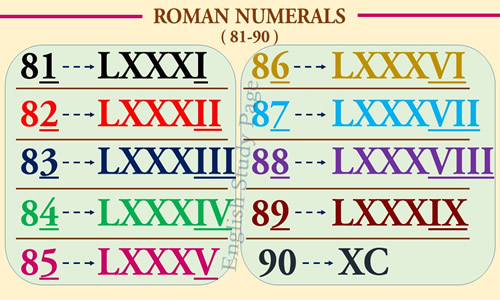From 91 to 100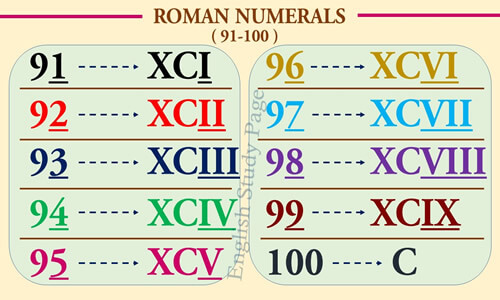From 10 to 100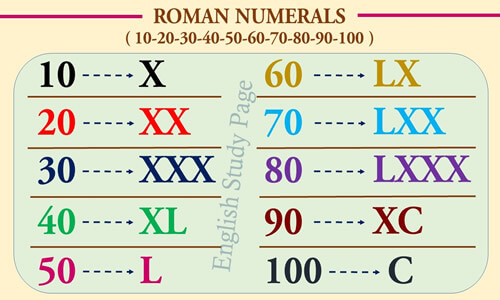From 100 to 1000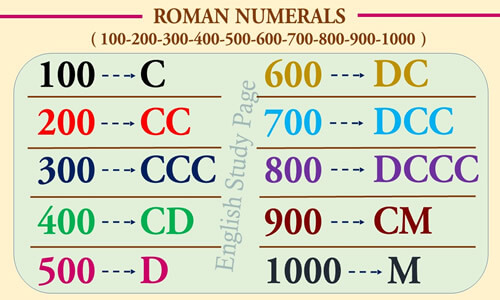From 1000 to 5000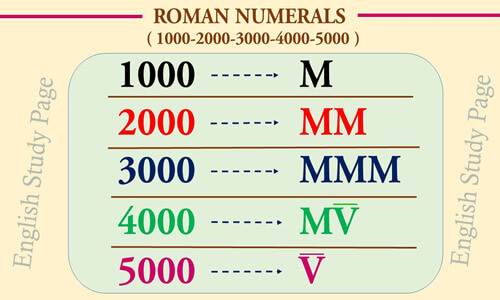From 10,000 to 1,000,000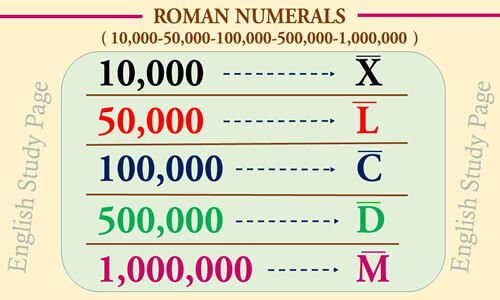From 5,000,000 to 1,000,000,0000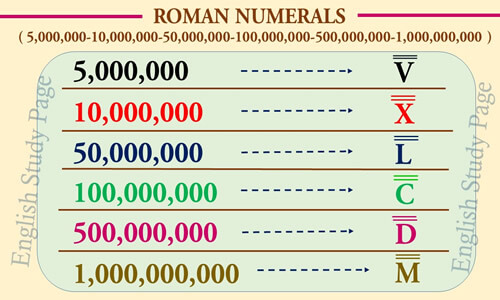Examples with Writing Roman Numerals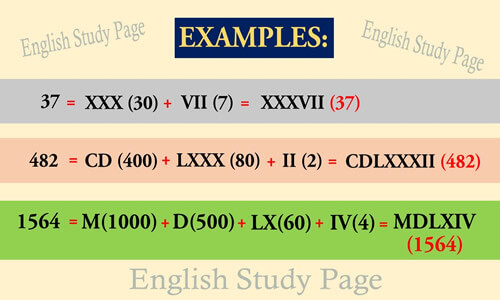### One thought on “Roman Numerals”

•January 18, 2018 at 6:48 am